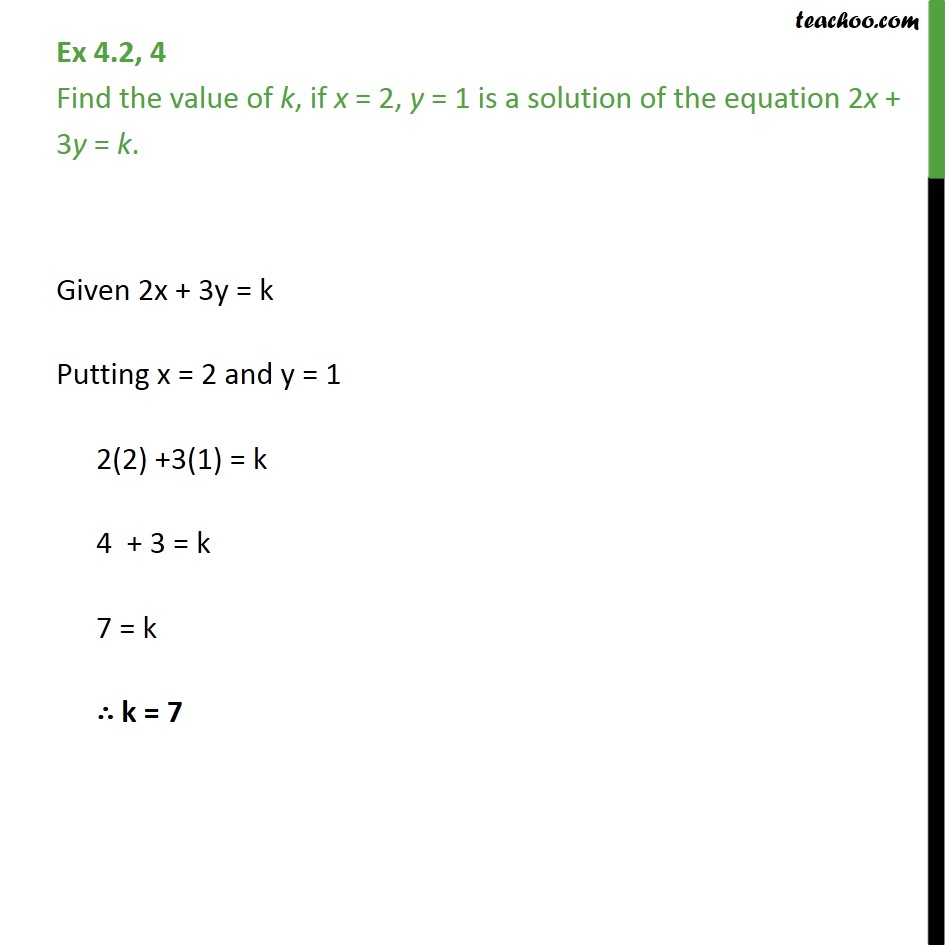1. Chapter 4 Class 9 Linear Equations in Two Variables
2. Concept wise
3. Solution of linear equation

Transcript

Ex 4.2, 4 - Chapter 4 Class 9 Linear Equations in Two Variables - NCERT Find the value of k, if x = 2, y = 1 is a solution of the equation 2x + 3y = k. Given 2x + 3y = k Putting x = 2 and y = 1 2(2) +3(1) = k 4 + 3 = k 7 = k ∴ k = 7

Solution of linear equation